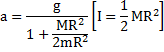# A uniform disk of massand radiusis mounted on a fixed horizontal axis. A block of masshangs from a mass less string that is wrapped around the rim of the disk. The magnitude of the acceleration of the falling blockis a)b)c)d)## Question ID - 52975 :- A uniform disk of massand radiusis mounted on a fixed horizontal axis. A block of masshangs from a mass less string that is wrapped around the rim of the disk. The magnitude of the acceleration of the falling blockis a)b)c)d)3537

 (b) UsingA straight rod of lengthhas one of its ends at the origin and the other at. If the mass per unit length of the rod is given bywhereis constant, where is its mass centre? a)b)c)d)